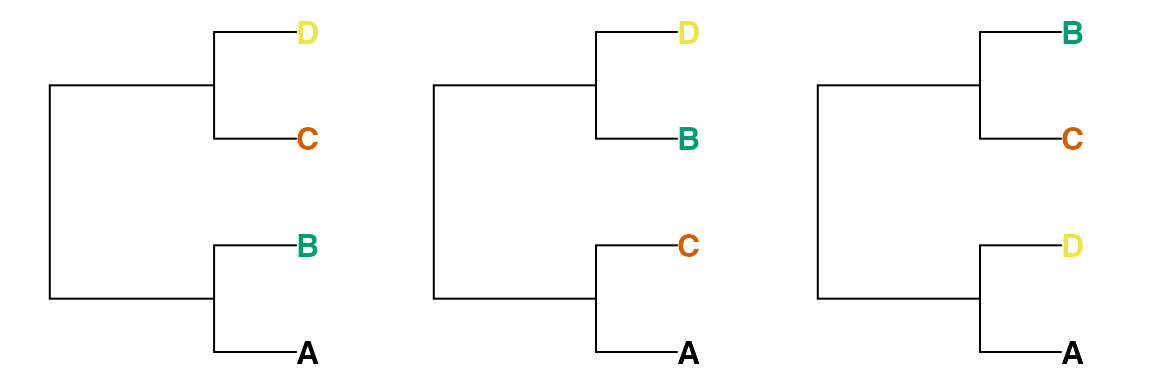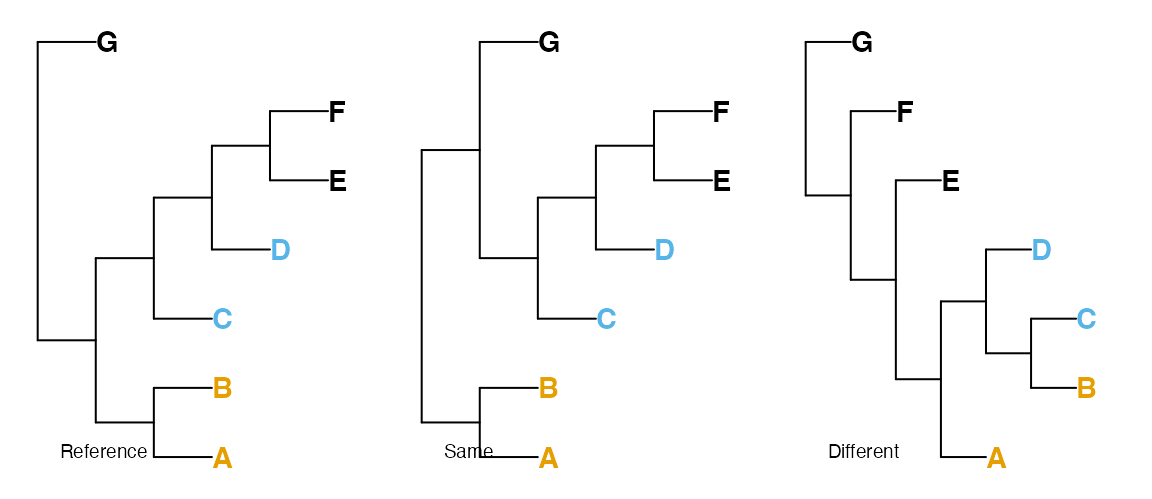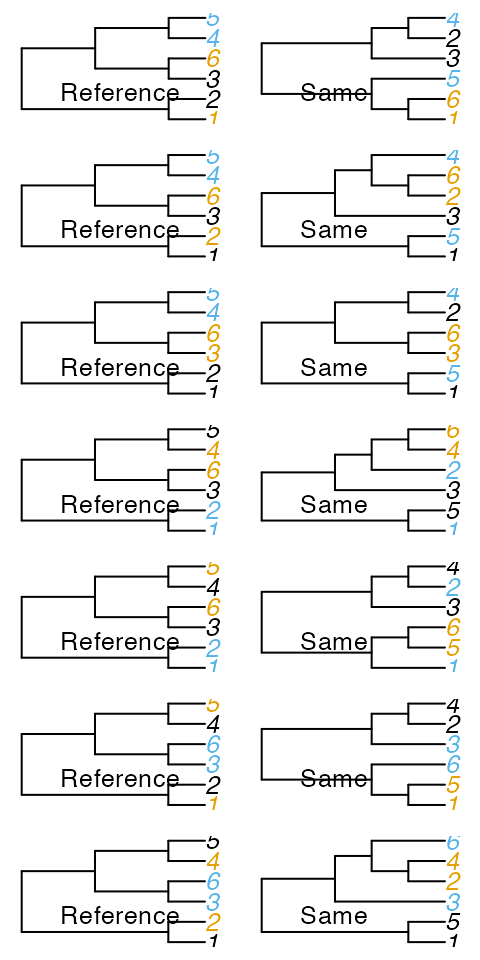## Partition distances

The Robinson-Foulds (RF or ‘partition’) metric (Robinson & Foulds, 1981; Steel & Penny, 1993) measures the symmetric difference between two trees by adding the number of splits (i.e. groupings) that are present in tree A (but not tree B) to the number of splits present in tree B (but not tree A).

It is most useful when the trees to be compared are very similar; it has a low range of integer values, and a low maximum value, limiting its ability to distinguish between trees (Steel & Penny, 1993); and it treats all splits as equivalent, even though some are more informative than others. Various other artefacts and biases limit its performance against a suite of real-world benchmarks (Smith, 2020, 2022). These shortcomings can largely be mitigated through generalizations of the Robinson-Foulds distance (see R package ‘TreeDist’), but the complementary perspective on tree similarity offered by a quartet-centred approach can be illuminating.

## Quartet distances

Instead of partitions, symmetric differences can be measured by counting the number of four-taxon statements (quartets) that differ between two trees (Day, 1986; Estabrook, McMorris, & Meacham, 1985).

For any four tips A, B, C and D, a split on a bifurcating tree will separate tip A and either B, C or D from the other two tips. That is to say, removing all other tips from the tree will leave one of these three trees:Thus two of the random trees below share the quartet (A, B), (C, D), whereas the third does not; these four tips are divided into (A, D), (B, C).There are $$\binom{n}{4}$$ groups of four taxa in a tree with $$n$$ tips; for each of these groups, one of the three trees above will be consistent with a given tree. As such, two identical trees will have a quartet distance of 0, and a random pair of trees will have an expected $$\binom{n}{4} / 3$$ quartets in common. Because quartets are not independent of one another, no pair of trees with six or more tips can have all $$\binom{n}{4}$$ quartets in common (Steel & Penny, 1993).

Properties of the quartet distance are explored fully in Steel & Penny (1993). As quartet distances of 1 can only be accomplished for small trees (five or fewer leaves; see below), it is perhaps more appropriate to consider whether or not trees are more dissimilar than a pair of random trees, whose distance will be, on average, $$\frac{2}{3}$$. (Data from real trees, and comparisons with expected values of other metrics, are available (here)[https://ms609.github.io/TreeDistData/articles/09-expected-similarity.html].)

## Normalization

Whereas counting quartets is simple, accounting for resolution is not. Two trees will have few quartet statements in common if they are well resolved and differ in many details; or if they are poorly resolved but in perfect agreement. As such, it is important to normalize quartet distances in a meaningful fashion. A number of normalizations have been proposed (Day, 1986; Estabrook et al., 1985); arguably the most appropriate is the Symmetric Quartet Divergence (Smith, 2019), which represents the total number of quartets unique to each tree normalized against the total number of quartets that could have been resolved. The SimilarityMetrics() documentation page gives further details.

## Asymmetric differences

Metric distances are necessarily symmetric – that is, the distance from tree A to tree B equals the distance from B to A. This behaviour is not necessarily desirable when one tree represents a known ‘reference’ – such as a tree validated by independent data, or a tree used to simulate data in order to test phylogenetic reconstruction techniques.

In such cases, a tree might be evaluated according to the likelihood that a randomly chosen quartet is resolved correctly by the tree, where an uncertain resolution in either the reference or comparison tree is taken as having a 1/3 chance of being correct (Asher & Smith forthcoming). More details are given at the SimilarityMetrics() documentation page.

### Quartet similarity in a pair of random trees

On average, $$\frac{1}{3}$$ of the quartets resolved in a pair of random trees will match. This is because there are three quartets involving any set of four tips, each of which is equally likely to occur on a truly random tree.

The below code calculates the mean proportion of matching quartets between 10 random trees (90 pairs) with 4 to 20 leaves, and the corresponding standard deviation.

round(vapply(4:20, function (nTip) {
trees <- lapply(rep(nTip, 10), TreeTools::RandomTree)
s <- ManyToManyQuartetAgreement(trees)[, , 's']
results <- s[lower.tri(s)] / choose(nTip, 4)
c(mean(results), sd(results))
}, c(mean = 0, sd = 0)), 3)
##       [,1]  [,2]  [,3]  [,4]  [,5]  [,6]  [,7]  [,8]  [,9] [,10] [,11] [,12]
## mean 0.311 0.378 0.311 0.367 0.347 0.317 0.334 0.359 0.346 0.329 0.343 0.339
## sd   0.468 0.257 0.194 0.157 0.112 0.087 0.092 0.106 0.080 0.061 0.066 0.049
##      [,13] [,14] [,15] [,16] [,17]
## mean 0.335  0.34 0.344 0.340 0.339
## sd   0.044  0.06 0.048 0.045 0.029

## Independence

One possible criticism of the quartet distance is that not all individual quartet statements are independent. For example, the quartet statements AB | CD and AB | CE together imply AB | DE. A simple count of identical quartets therefore includes some redundant information. This prevents a straightforward information theoretic interpretation of the quartet distance.

## Minimum quartet similarity

As a related phenomenon, when there are six or more tips in a bifurcating tree, some quartets are necessarily shared between trees.

Consider the tree:

tree_a <- ape::read.tree(text = "((1, 2), (3, (4, 5)));")The only trees with no quartets in common with Tree A are symmetric with

tree_b <- ape::read.tree(text = "((1, 5), (3, (2, 4)));")Now create Tree C by adding a 6th tip as a sister to tip 3 on Tree A.

tree_c <- ape::read.tree(text="((1, 2), ((3, 6), (4, 5)));")There’s nowhere to add tip 6 to Tree B without creating a quartet that exists in Tree C.As such, the minimum possible quartet similarity is non-zero, and becomes increasingly difficult to compute as the number of leaves rises. This fact increases the value of comparing low quartet similarity scores to the expected similarity of a pair of random trees (i.e. $$\frac{1}{3}$$), rather than to zero.

Day, W. H. E. (1986). Analysis of quartet dissimilarity measures between undirected phylogenetic trees. Systematic Biology, 35(3), 325–333. doi: 10.1093/sysbio/35.3.325
Estabrook, G. F., McMorris, F. R., & Meacham, C. A. (1985). Comparison of undirected phylogenetic trees based on subtrees of four evolutionary units. Systematic Zoology, 34(2), 193–200. doi: 10.2307/2413326
Robinson, D. F., & Foulds, L. R. (1981). Comparison of phylogenetic trees. Mathematical Biosciences, 53(1-2), 131–147. doi: 10.1016/0025-5564(81)90043-2
Smith, M. R. (2019). Bayesian and parsimony approaches reconstruct informative trees from simulated morphological datasets. Biology Letters, 15, 20180632. doi: 10.1098/rsbl.2018.0632
Smith, M. R. (2020). Information theoretic Generalized Robinson-Foulds metrics for comparing phylogenetic trees. Bioinformatics, 36(20), 5007–5013. doi: 10.1093/bioinformatics/btaa614
Smith, M. R. (2022). Robust analysis of phylogenetic tree space. Systematic Biology, 71(5), 1255–1270. doi: 10.1093/sysbio/syab100
Steel, M. A., & Penny, D. (1993). Distributions of tree comparison metrics—some new results. Systematic Biology, 42(2), 126–141. doi: 10.1093/sysbio/42.2.126